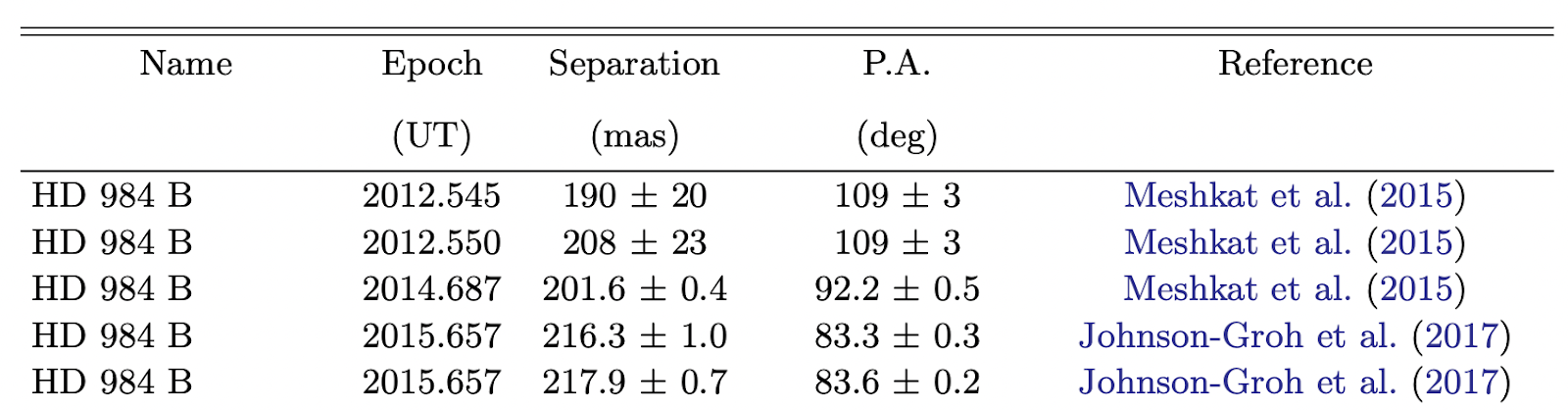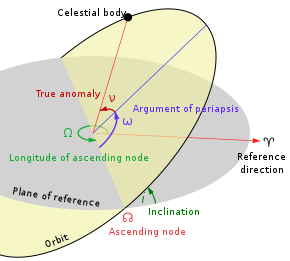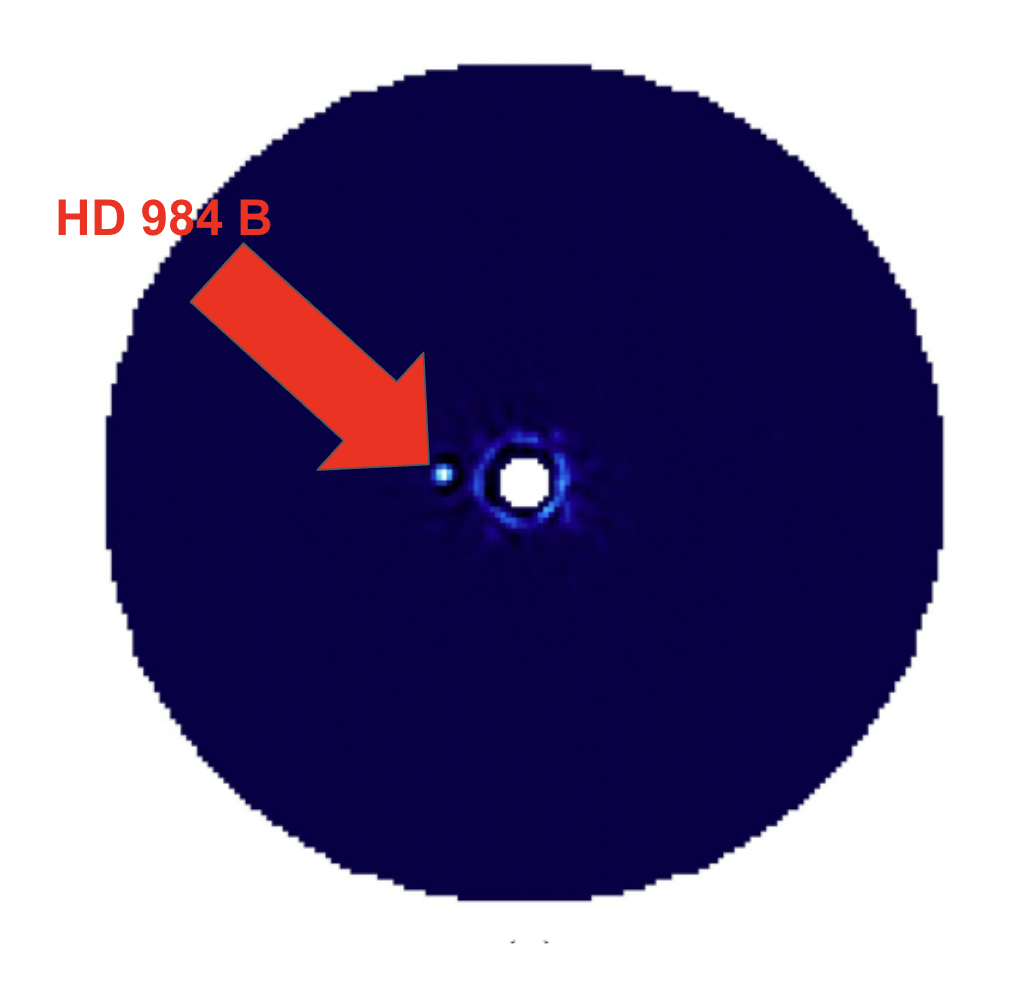# Learning more about exoplanet orbits using orbitize!

Orbitize! is a software used in the scientific community to fit orbits of directly imaged exoplanets. Sounds cool, but what does it mean?

Most of the exoplanets we directly image are located far away from their host star, which means they have very long periods. Since we can’t continuously observe these objects, we often obtain their “astrometry” – their x and y (or r and$\theta$ if you’re working in polar coordinates) positions relative to the star at each time of observation, along with their uncertainties. An astrometry table example is shown in Figure 1.Figure 1. Table from Bowler et al. 2020 of the r and   (called separation and position angle (PA)) of the companion to the star HD 984 called HD 984 B at different epochs.

The positions are useful to us because we can use them to estimate the orbit of the planets in 3D – are the orbits eccentric, or more circular? What is the semi-major axis of the orbit? Is it an inclined orbit relative to us here on Earth? Since the direct imaging method is relatively new, the community had been in need of an open source code that could help us answer these questions and better understand the orbits of these exoplanets. We want to learn more about exoplanet orbits because they can tell us a lot about how the planetary systems formed and how they will evolve over time.Figure 2. The 6 orbital elements that describe a 3D orbit are the semi-major axis (a), the eccentricity (e), the inclination (i), the longitude of ascending node (), the argument of periastron () and the true anomaly (). This drawing has a different reference direction than the one used in exoplanet science (our reference direction is going up, usually).

Caltech graduate student Sarah Blunt led an effort to write an orbit fitting code in Python that is open source and pretty straightforward to use, called orbitize! (yes, with the exclamation point!). It has since become a standard in the exoplanet direct imaging community. This code allows you to use the planet positions to estimate the orbital parameters of the planet, using two main fitting algorithms: Orbits For The Impatient (OFTI) and Markov-Chain Monte Carlo (MCMC). All of the 6 orbital parameters are shown on Figure 2.Figure 3. HD 984 B seen using GPI (from Johnson-Groh et al 2017).

In today’s post, we will learn how to download and use orbitize! with the MCMC functionality to fit for the possible orbits of the exoplanet HD 984 B (discovered by Meshkat et al 2015), shown in Figure 3. (NOTE: this tutorial assumes that you have Python 3 installed in your computer, along with Python packages NumPy and Matplotlib – if you do not have those installed, please click the links to learn how to install them).

We will need to properly format our astrometry so orbitize! can read it. Orbitize! Takes a CSV file (which can be written on Google Sheets or Excel) where the columns, respectively, must be:

• “epoch” – this is the time of your astrometry observation, in Modified Julian Date (MJD). You can use an online converter to convert our regular dates to MJD.
• “object” – this refers to which object your astrometry is for. Here, since we are using astrometry from the planet, we always put “1” values in this corner.
• “sep” – this is the separation of your object to the host star, in milliarcseconds (mas).
• “sep_err” – this is the uncertainty of your object’s separation in mas.
• “pa” – this is the position angle of your planet, in degrees.
• “pa_err” – this is the position angle uncertainty of your planet, in degrees.

Once you have your data table formatted, you must download it as a CSV file.

Figure 4 shows an example on how to format this data, for HD 984 B. In order to facilitate this tutorial, I have uploaded this CSV file to GitHub, so you can access it from there by clicking this link.

Figure 4. Astrometry for HD 984 B that will be used for orbitize!

Now that you have the file all set to go, we will move over to a Jupyter notebook for the rest of the tutorial.